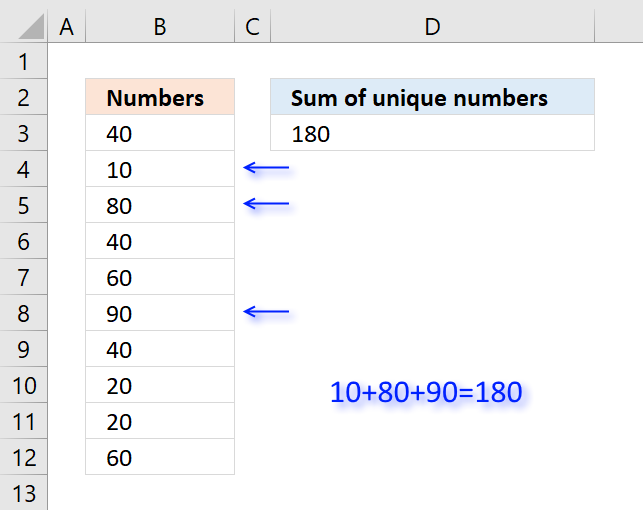Author: Oscar Cronquist Article last updated on November 15, 2018The formula in cell D3 adds all unique numbers in cell range B3:B12 and returns the total. Unique values are all values occurring only once in the list.

Formula in cell D3:

=SUMPRODUCT((COUNTIF(B3:B12,B3:B12)=1)*B3:B12)

### Explaining formula in cell D3

#### Step 1 - Count occurrence of each value in cell range

The COUNTIF function is an extremely useful function, this time I am counting how many times each value shows up in the cell range.

COUNTIF(B3:B12,B3:B12)

becomes

COUNTIF({40; 10; 80; 40; 60; 90; 40; 20; 20; 60},{40; 10; 80; 40; 60; 90; 40; 20; 20; 60})

and returns

{3;1;1;3;2;1;3;2;2;2}

#### Step 2 - Check if value is unique

The equal sign checks if number is equal to 1.

COUNTIF(B3:B12,B3:B12)=1

becomes

{3;1;1;3;2;1;3;2;2;2}=1

and returns

{FALSE; TRUE; TRUE; FALSE; FALSE; TRUE; FALSE; FALSE; FALSE; FALSE}

#### Step 3 - Multiply with cell range

The boolean values in the array show which numbers to include in sum. FALSE is the same as 0 (zero) and TRUE is equivalebt to 1. The parentheses make sure that the comparison is calculated before multiplying.

(COUNTIF(B3:B12,B3:B12)=1)*B3:B12

becomes

{FALSE; TRUE; TRUE; FALSE; FALSE; TRUE; FALSE; FALSE; FALSE; FALSE}*B3:B12

becomes

{FALSE; TRUE; TRUE; FALSE; FALSE; TRUE; FALSE; FALSE; FALSE; FALSE}*{3;1;1;3;2;1;3;2;2;2}

and returns

{0;10;80;0;0;90;0;0;0;0}

#### Step 4 - Sum numbers

The SUMPRODUCT function now simply adds the numbers and returns the total.

SUMPRODUCT((COUNTIF(B3:B12,B3:B12)=1)*B3:B12)

becomes

SUMPRODUCT({0;10;80;0;0;90;0;0;0;0})

and returns 180 in cell D3.

Why use the SUMPRODUCT function and not the SUM function? You don't need to enter this formula as an array formula if you use the SUMPRODUCT function.